# Pre-Algebra : Product Rule of Exponents

## Example Questions

### Example Question #21 : Product Rule Of Exponents

Simplify: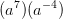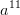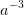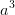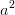Explanation:

When you multiply exponents with the same base, you add the exponents: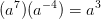### Example Question #22 : Product Rule Of Exponents

Simplify: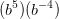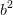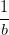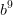Explanation:

When you multiply exponents with the same base, you add the exponents: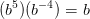### Example Question #23 : Product Rule Of Exponents

Simplify: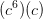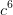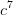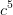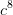Explanation:

When you multiply exponents with the same base, you add the exponents: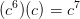### Example Question #22 : Product Rule Of Exponents

Simplify: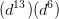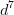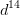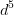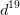Explanation:

When you multiply exponents with the same base, you add the exponents: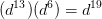### Example Question #23 : Product Rule Of Exponents

Simplify: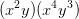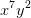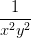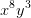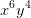Explanation:

When you multiply exponents with the same base, you add the exponents: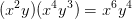### Example Question #24 : Product Rule Of Exponents

Simplify: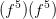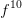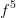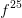Explanation:

When you multiply exponents with the same base, you add the exponents: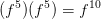### Example Question #25 : Product Rule Of Exponents

Simplify: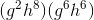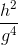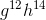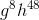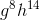Explanation:

When you multiply exponents with the same base, you add the exponents: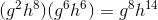### Example Question #26 : Product Rule Of Exponents

Simplify: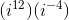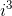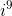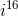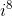Explanation:

When you multiply exponents with the same base, you add the exponents: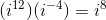### Example Question #27 : Product Rule Of Exponents

Simplify: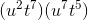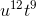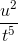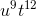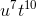Explanation:

When you multiply exponents with the same base, you add the exponents: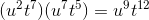### Example Question #21 : Product Rule Of Exponents

Simplify: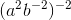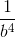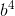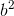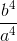Explanation:

According to the power rule for exponents, if a quantity is raised to a certain exponent, the powers of the inner quantity can be multiplied with the power outside the parentheses.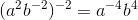Eliminate the negative exponent by the following rule: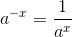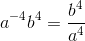### All Pre-Algebra Resources